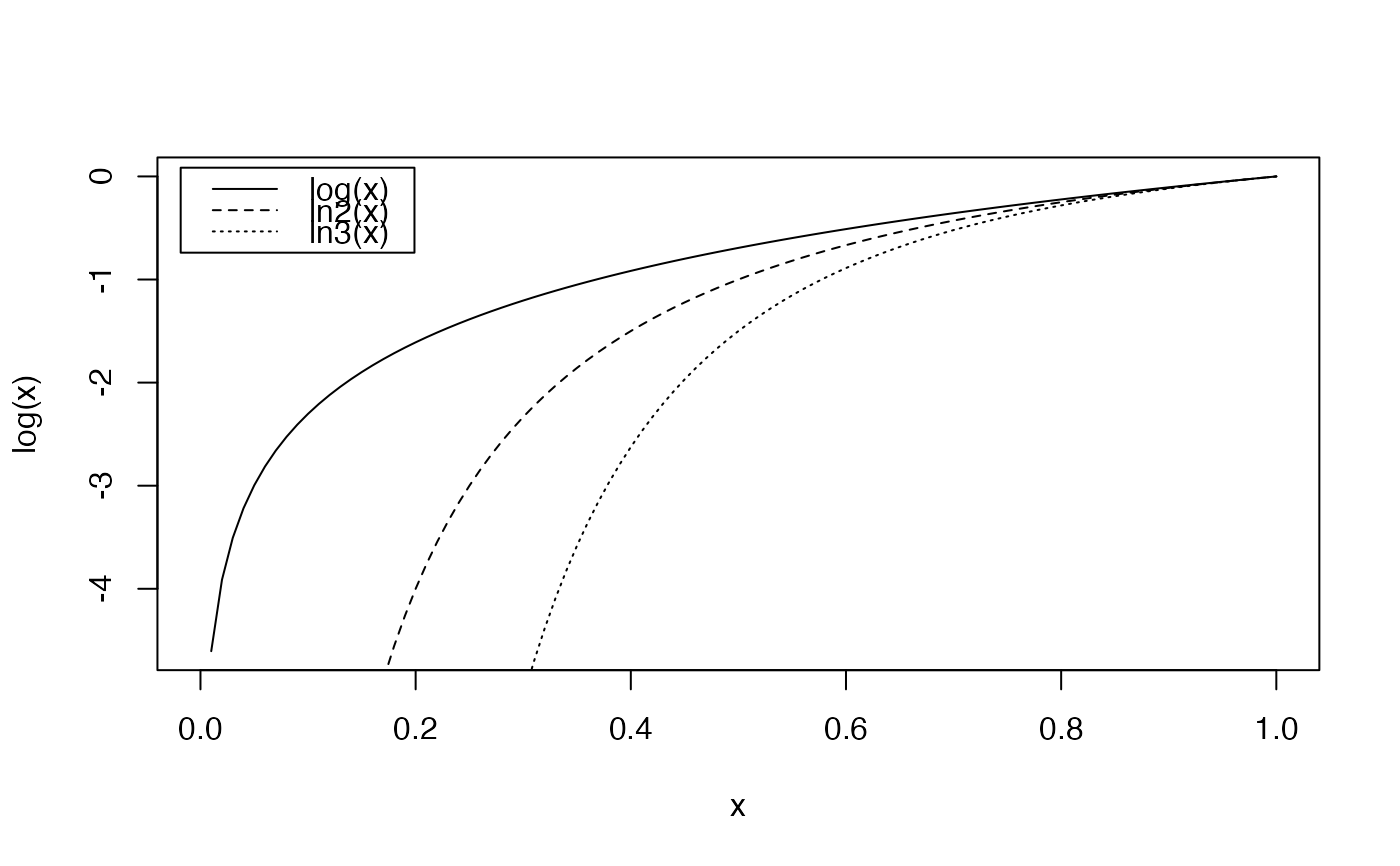Calculates the deformed logarithm of order $$q$$.

lnq(x, q)
lnq.CommunityProfile(Profile)

## Arguments

x

A numeric vector.

Profile

A CommunityProfile.

q

A number.

## Details

The deformed logarithm is defined as $$\ln_q{x}=\frac{(x^{(1-q)}-1)}{(1-q)}$$.

The shape of the deformed logarithm is similar to that of the regular one. $$\ln_1{x}=\log{x}$$.

For $$q>1$$, $$\ln_q{(+\infty)}=\frac{1}{(q-1)}$$.

lnq.CommunityProfile calculates the deformed logarithm of a CommunityProfile. Its $x item (the order of dversity) is kept unchanged whilst other items are set to their logarithm of order $x. Thus, a diversity profile is transformed into an entropy profile.

## Value

A vector of the same length as x containing the transformed values or a CommunityProfile.

expq
curve(log(x), 0, 1, lty=1)